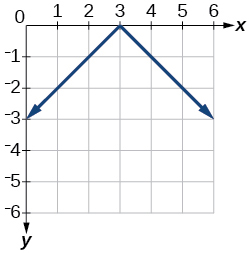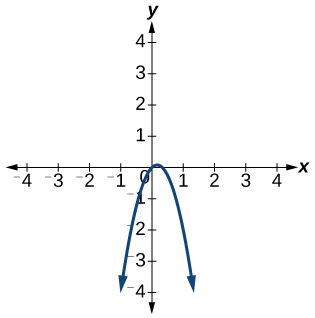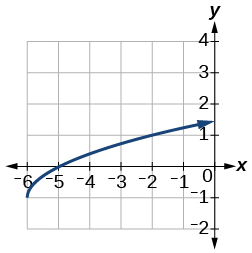# 1.7 Inverse functions  (Page 9/10)

 Page 9 / 10

$f\left(x\right)=|x-5|$

$f\left(x\right)=-|x-3|$$f\left(x\right)=|2x-4|$

For the following exercises, solve the absolute value equation.

$|x+4|=18$

$|\frac{1}{3}x+5|=|\frac{3}{4}x-2|$

For the following exercises, solve the inequality and express the solution using interval notation.

$|3x-2|<7$

$\left(-\frac{5}{3},3\right)$

$|\frac{1}{3}x-2|\le 7$

## Inverse Functions

For the following exercises, find for each function.

$f\left(x\right)=9+10x$

$f\left(x\right)=\frac{x}{x+2}$

${f}^{-1}\left(x\right)=\frac{-2x}{x-1}$

For the following exercise, find a domain on which the function is one-to-one and non-decreasing. Write the domain in interval notation. Then find the inverse of restricted to that domain.

$f\left(x\right)={x}^{2}+1$

Given $f\left(x\right)={x}^{3}-5$ and $g\left(x\right)=\sqrt{x+5}:$

1. Find and $g\left(f\left(x\right)\right).$
2. What does the answer tell us about the relationship between $f\left(x\right)$ and $g\left(x\right)?$
1. and $g\left(f\left(x\right)\right)=x.$
2. This tells us that $f$ and $g$ are inverse functions

For the following exercises, use a graphing utility to determine whether each function is one-to-one.

$f\left(x\right)=\frac{1}{x}$

The function is one-to-one.$f\left(x\right)=-3{x}^{2}+x$

The function is not one-to-one.If $f\left(5\right)=2,$ find ${f}^{-1}\left(2\right).$

$5$

If $f\left(1\right)=4,$ find ${f}^{-1}\left(4\right).$

## Practice test

For the following exercises, determine whether each of the following relations is a function.

$y=2x+8$

The relation is a function.

$\left\{\left(2,1\right),\left(3,2\right),\left(-1,1\right),\left(0,-2\right)\right\}$

For the following exercises, evaluate the function $\text{\hspace{0.17em}}f\left(x\right)=-3{x}^{2}+2x\text{\hspace{0.17em}}$ at the given input.

$f\left(-2\right)$

−16

$\text{\hspace{0.17em}}f\left(a\right)\text{\hspace{0.17em}}$

Show that the function $\text{\hspace{0.17em}}f\left(x\right)=-2{\left(x-1\right)}^{2}+3\text{\hspace{0.17em}}$ is not one-to-one.

The graph is a parabola and the graph fails the horizontal line test.

Write the domain of the function $\text{\hspace{0.17em}}f\left(x\right)=\sqrt{3-x}\text{\hspace{0.17em}}$ in interval notation.

Given $\text{\hspace{0.17em}}f\left(x\right)=2{x}^{2}-5x,\text{\hspace{0.17em}}$ find $\text{\hspace{0.17em}}f\left(a+1\right)-f\left(1\right).$

$2{a}^{2}-a$

Graph the function

Find the average rate of change of the function $\text{\hspace{0.17em}}f\left(x\right)=3-2{x}^{2}+x\text{\hspace{0.17em}}$ by finding $\text{\hspace{0.17em}}\frac{f\left(b\right)-f\left(a\right)}{b-a}.$

$-2\left(a+b\right)+1$

For the following exercises, use the functions to find the composite functions.

$\left(g\circ f\right)\left(x\right)$

$\left(g\circ f\right)\left(1\right)$

$\sqrt{2}$

Express $\text{\hspace{0.17em}}H\left(x\right)=\sqrt{5{x}^{2}-3x}\text{\hspace{0.17em}}$ as a composition of two functions, $\text{\hspace{0.17em}}f\text{\hspace{0.17em}}$ and $\text{\hspace{0.17em}}g,\text{\hspace{0.17em}}$ where $\text{\hspace{0.17em}}\left(f\circ g\right)\left(x\right)=H\left(x\right).$

For the following exercises, graph the functions by translating, stretching, and/or compressing a toolkit function.

$f\left(x\right)=\sqrt{x+6}-1$$f\left(x\right)=\frac{1}{x+2}-1$

For the following exercises, determine whether the functions are even, odd, or neither.

$f\left(x\right)=-\frac{5}{{x}^{2}}+9{x}^{6}$

$\text{even}$

$f\left(x\right)=-\frac{5}{{x}^{3}}+9{x}^{5}$

$f\left(x\right)=\frac{1}{x}$

$\text{odd}$

Graph the absolute value function $\text{\hspace{0.17em}}f\left(x\right)=-2|x-1|+3.$

Solve $|2x-3|=17.$

$x=-7\text{\hspace{0.17em}}$ and $\text{\hspace{0.17em}}x=10$

Solve $\text{\hspace{0.17em}}-|\frac{1}{3}x-3|\ge 17.\text{\hspace{0.17em}}$ Express the solution in interval notation.

For the following exercises, find the inverse of the function.

$f\left(x\right)=3x-5$

${f}^{-1}\left(x\right)=\frac{x+5}{3}$

$f\left(x\right)=\frac{4}{x+7}$

For the following exercises, use the graph of $\text{\hspace{0.17em}}g\text{\hspace{0.17em}}$ shown in [link] .

On what intervals is the function increasing?

On what intervals is the function decreasing?

Approximate the local minimum of the function. Express the answer as an ordered pair.

$\left(1.1,-0.9\right)$

Approximate the local maximum of the function. Express the answer as an ordered pair.

For the following exercises, use the graph of the piecewise function shown in [link] .

Find $\text{\hspace{0.17em}}f\left(2\right).$

$f\left(2\right)=2$

Find $\text{\hspace{0.17em}}f\left(-2\right).$

Write an equation for the piecewise function.

$f\left(x\right)=\left\{\begin{array}{c}|x|\text{\hspace{0.17em}}\text{\hspace{0.17em}}\text{\hspace{0.17em}}\text{if}\text{\hspace{0.17em}}\text{\hspace{0.17em}}x\le 2\\ 3\text{\hspace{0.17em}}\text{\hspace{0.17em}}\text{\hspace{0.17em}}\text{\hspace{0.17em}}\text{\hspace{0.17em}}\text{if}\text{\hspace{0.17em}}\text{\hspace{0.17em}}x>2\end{array}$

For the following exercises, use the values listed in [link] .

 $x$ $F\left(x\right)$ 0 1 1 3 2 5 3 7 4 9 5 11 6 13 7 15 8 17

Find $\text{\hspace{0.17em}}F\left(6\right).$

Solve the equation $\text{\hspace{0.17em}}F\left(x\right)=5.$

$x=2$

Is the graph increasing or decreasing on its domain?

Is the function represented by the graph one-to-one?

yes

Find $\text{\hspace{0.17em}}{F}^{-1}\left(15\right).$

Given $\text{\hspace{0.17em}}f\left(x\right)=-2x+11,\text{\hspace{0.17em}}$ find $\text{\hspace{0.17em}}{f}^{-1}\left(x\right).$

${f}^{-1}\left(x\right)=-\frac{x-11}{2}$

how to prroved cos⁴x-sin⁴x= cos²x-sin²x are equal
Don't think that you can.
Elliott
how do you provided cos⁴x-sin⁴x = cos²x-sin²x are equal
What are the question marks for?
Elliott
Someone should please solve it for me Add 2over ×+3 +y-4 over 5 simplify (×+a)with square root of two -×root 2 all over a multiply 1over ×-y{(×-y)(×+y)} over ×y
For the first question, I got (3y-2)/15 Second one, I got Root 2 Third one, I got 1/(y to the fourth power) I dont if it's right cause I can barely understand the question.
Is under distribute property, inverse function, algebra and addition and multiplication function; so is a combined question
Abena
find the equation of the line if m=3, and b=-2
graph the following linear equation using intercepts method. 2x+y=4
Ashley
how
Wargod
what?
John
ok, one moment
UriEl
how do I post your graph for you?
UriEl
it won't let me send an image?
UriEl
also for the first one... y=mx+b so.... y=3x-2
UriEl
y=mx+b you were already given the 'm' and 'b'. so.. y=3x-2
Tommy
Please were did you get y=mx+b from
Abena
y=mx+b is the formula of a straight line. where m = the slope & b = where the line crosses the y-axis. In this case, being that the "m" and "b", are given, all you have to do is plug them into the formula to complete the equation.
Tommy
thanks Tommy
Nimo
"7"has an open circle and "10"has a filled in circle who can I have a set builder notation
x=-b+_Гb2-(4ac) ______________ 2a
I've run into this: x = r*cos(angle1 + angle2) Which expands to: x = r(cos(angle1)*cos(angle2) - sin(angle1)*sin(angle2)) The r value confuses me here, because distributing it makes: (r*cos(angle2))(cos(angle1) - (r*sin(angle2))(sin(angle1)) How does this make sense? Why does the r distribute once
so good
abdikarin
this is an identity when 2 adding two angles within a cosine. it's called the cosine sum formula. there is also a different formula when cosine has an angle minus another angle it's called the sum and difference formulas and they are under any list of trig identities
strategies to form the general term
carlmark
consider r(a+b) = ra + rb. The a and b are the trig identity.
Mike
How can you tell what type of parent function a graph is ?
generally by how the graph looks and understanding what the base parent functions look like and perform on a graph
William
if you have a graphed line, you can have an idea by how the directions of the line turns, i.e. negative, positive, zero
William
y=x will obviously be a straight line with a zero slope
William
y=x^2 will have a parabolic line opening to positive infinity on both sides of the y axis vice versa with y=-x^2 you'll have both ends of the parabolic line pointing downward heading to negative infinity on both sides of the y axis
William
y=x will be a straight line, but it will have a slope of one. Remember, if y=1 then x=1, so for every unit you rise you move over positively one unit. To get a straight line with a slope of 0, set y=1 or any integer.
Aaron
yes, correction on my end, I meant slope of 1 instead of slope of 0
William
what is f(x)=
I don't understand
Joe
Typically a function 'f' will take 'x' as input, and produce 'y' as output. As 'f(x)=y'. According to Google, "The range of a function is the complete set of all possible resulting values of the dependent variable (y, usually), after we have substituted the domain."
Thomas
Sorry, I don't know where the "Â"s came from. They shouldn't be there. Just ignore them. :-)
Thomas
Darius
Thanks.
Thomas
Â
Thomas
It is the Â that should not be there. It doesn't seem to show if encloses in quotation marks. "Â" or 'Â' ... Â
Thomas
Now it shows, go figure?
Thomas
what is this?
i do not understand anything
unknown
lol...it gets better
Darius
I've been struggling so much through all of this. my final is in four weeks 😭
Tiffany
this book is an excellent resource! have you guys ever looked at the online tutoring? there's one that is called "That Tutor Guy" and he goes over a lot of the concepts
Darius
thank you I have heard of him. I should check him out.
Tiffany
is there any question in particular?
Joe
I have always struggled with math. I get lost really easy, if you have any advice for that, it would help tremendously.
Tiffany
Sure, are you in high school or college?
Darius
Hi, apologies for the delayed response. I'm in college.
Tiffany
how to solve polynomial using a calculator
So a horizontal compression by factor of 1/2 is the same as a horizontal stretch by a factor of 2, right?
The center is at (3,4) a focus is at (3,-1), and the lenght of the major axis is 26
The center is at (3,4) a focus is at (3,-1) and the lenght of the major axis is 26 what will be the answer?
Rima
I done know
Joe
What kind of answer is that😑?
Rima
I had just woken up when i got this message
Joe
Rima
i have a question.
Abdul
how do you find the real and complex roots of a polynomial?
Abdul
@abdul with delta maybe which is b(square)-4ac=result then the 1st root -b-radical delta over 2a and the 2nd root -b+radical delta over 2a. I am not sure if this was your question but check it up
Nare
This is the actual question: Find all roots(real and complex) of the polynomial f(x)=6x^3 + x^2 - 4x + 1
Abdul
@Nare please let me know if you can solve it.
Abdul
I have a question
juweeriya
hello guys I'm new here? will you happy with me
mustapha
The average annual population increase of a pack of wolves is 25.
how do you find the period of a sine graph
Period =2π if there is a coefficient (b), just divide the coefficient by 2π to get the new period
Am
if not then how would I find it from a graph
Imani
by looking at the graph, find the distance between two consecutive maximum points (the highest points of the wave). so if the top of one wave is at point A (1,2) and the next top of the wave is at point B (6,2), then the period is 5, the difference of the x-coordinates.
Am
you could also do it with two consecutive minimum points or x-intercepts
Am
I will try that thank u
ImaniByBy Abishek DevarajByByByBy